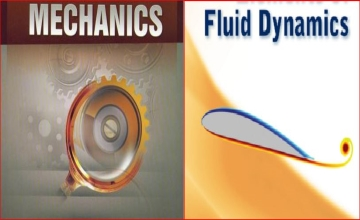# Mechanics and Fluid Dynamics for UPSC

• Home
• >
• Latest News
• >
• Mechanics and Fluid Dynamics for UPSC

#### Mechanics and Fluid Dynamics for UPSC

Posted by Ramanasri Nov 29 2020

Mechanics and Fluid Dynamics for UPSC

Ramanasri IAS Maths Optional is teaching Mechanics and Fluid Dynamics for UPSC. Ramanasri Sir is also teaching all the 13 Modules. For example from 1st Module Linear Algebra to Last i.e.,13th Module Mechanics & Fluid Dynamics. This Mechanics and Fluid Dynamics Module is Difficulty Level like Real Analysis, Statics, and Dynamics, Modern Algebra. In Ramanasri IAS Ramanasri Sir is delivering Best Lectures for these all four Modules in addition to the rest of 9 Modules. The rest of the 9 Modules 5 Modules are very easy like Vector Analysis, Complex Analysis, Linear Programming, Numerical Analysis and Computer Programming, Calculus.
Medium Level Modules are Ordinary Differential Equations, Partial Differential Equations, Analytical Geometry.

This Below we are giving Mechanics and Fluid Dynamics for UPSC Maths Syllabus. And also Mechanics and Fluid Dynamics for IFS or IFoS Maths Syllabus.

Mechanics and Fluid Dynamics for UPSC Maths Syllabus | IAS Maths Syllabus

Generalized coordinates; D’Alembert’s principle and Lagrange’s equations; Hamilton equations; Moment of inertia; Motion of rigid bodies in two dimensions. Equation of continuity; Euler’s equation of motion for Inviscid flow; Stream-lines, the path of a particle; Potential flow; Two-dimensional and asymmetric motion; Sources and sinks, vortex motion; Navier-Stokes Equation for a viscous fluid.

Mechanics and Fluid Dynamics for IFS Maths Syllabus | IFoS Maths Syllabus.

Generalized coordinates, constraints, holonomic and non-holonomic, systems. D’Alemebert’s principle and Lagrange’ equations, Hamilton equations, the moment of inertia, the motion of rigid bodies in two dimensions. Equation of continuity, Euler’s equation of motion for Inviscid flow, stream-lines, the path of a particle, potential flow, two-dimensional and axisymmetric motion, sources and sinks, vortex motion, flow past a cylinder and a sphere, method of images. Navier-Stokes equation for a viscous fluid.

Mechanics and Fluid Dynamics Common topics for UPSC Maths Syllabus | IAS Maths Syllabus & IFS or IFoS Maths Syllabus

Generalized coordinates; D’Alembert’s principle and Lagrange’s equations; Hamilton equations; Moment of inertia; Motion of rigid bodies in two dimensions. Equation of continuity; Euler’s equation of motion for Inviscid flow; Stream-lines, the path of a particle; Potential flow; Two-dimensional and asymmetric motion; Sources and sinks, vortex motion; Navier-Stokes Equation for a viscous fluid.

List of topics of Mechanics and Fluid Dynamics for ONLY UPSC Maths Syllabus | IAS Maths Syllabus

No topics

List of topics of Mechanics and Fluid Dynamics for ONLY IFS Maths Syllabus | IFoS Maths Syllabus

constraints, holonomic and non-holonomic, systems. flow past a cylinder and a sphere, method of images.

Click on this below links to know more details.

Click on this below links to know more details about RAMANASRI IAS YouTube Channel.

RAMANASRI IAS YouTube ChannelGmap Helper